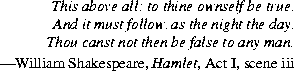Contents | Prev | Next | Index

# 20.4 The Class `java.lang.Boolean`

Objects of type `Boolean` represent primitive values of type `boolean`.

```public final class `Boolean` {
public static final Boolean `TRUE` = new Boolean(true);
public static final Boolean `FALSE` = new Boolean(false);
public `Boolean`(boolean value);
public `Boolean`(String s);
public String `toString`();
public boolean `equals`(Object obj);
public int `hashCode`();
public boolean `booleanValue`();
public static Boolean `valueOf`(String s);
public static boolean `getBoolean`(String name);
}
```

20.4.1 `public static final Boolean TRUE = new Boolean(true);`

The constant value of this field is a `Boolean` object corresponding to the primitive value `true`.

20.4.2 `public static final Boolean FALSE = new Boolean(false);`

The constant value of this field is a `Boolean` object corresponding to the primitive value `false`.

20.4.3 `public Boolean(boolean value)`

This constructor initializes a newly created `Boolean` object so that it represents the primitive value that is the argument.

20.4.4 `public Boolean(String s)`

This constructor initializes a newly created `Boolean` object so that it represents `true` if and only if the argument is not `null` and is equal, ignoring case, to the string `"true"`.

Examples:

```
`new` `Boolean("True")` produces a `Boolean` object that represents `true`.
`new` `Boolean("yes")` produces a `Boolean` object that represents `false`.
```

20.4.5 `public String toString()`

If this `Boolean` object represents `true`, a string equal to `"true"` is returned. If this `Boolean` object represents `false`, a string equal to `"false"` is returned.

Overrides the `toString` method of `Object` (§20.1.2).

20.4.6 `public boolean equals(Object obj)`

The result is `true` if and only if the argument is not `null` and is a `Boolean` object that represents the same `boolean` value as this `Boolean` object.

Overrides the `equals` method of `Object` (§20.1.3).

20.4.7 `public int hashCode()`

If this `Boolean` object represents `true`, the integer `1231` is returned. If this `Boolean` object represents `false`, the integer `1237` is returned.

Overrides the `hashCode` method of `Object` (§20.1.4).

20.4.8 `public boolean booleanValue()`

The primitive `boolean` value represented by this `Boolean` object is returned.

20.4.9 `public static boolean valueOf(String s)`

The result is `true` if and only if the argument is not `null` and is equal, ignoring case, to the string `"true"`.

Example: `Boolean.valueOf("True")` returns `true`.

Example: `Boolean.valueOf("yes")` returns `false`.

20.4.10 `public static boolean getBoolean(String name)`

The result is `true` if and only if the value of the system property (§20.18.9) named by the argument is equal, ignoring case, to the string `"true"`.Java Language Specification (HTML generated by Suzette Pelouch on February 24, 1998)
Copyright © 1996 Sun Microsystems, Inc. All rights reserved
Please send any comments or corrections to [email protected]